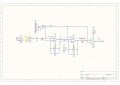# Help needed with Low/High cutoff filter modification of RF Doppler circuit for higher speed reading

#### fe7565

Joined Aug 13, 2016
9I am looking at the attached circuit that uses a CDM-234 Doppler sensor with a very low voltage output. The attached circuit is setup to read a bandwidth of around between 0-115 FPS speed and I want it to read between 300-1,300 FPS. I am hoping to modify the circuit by changing the values of resistors and capacitors for Opamp 1 and Opamp 2 (see below).

Current High cutoff is about 3 hz and the Low cutoff is about 999 hz. The new High Cutoff should be 15 Khz and Low cutoff 65 khz.

High cutoff opamp1: C18=10uF R17= 4.7Kohm => new values C18=1nF R17=10Kohm
Low cutoff opamp1: C23=270pF R20=590Kohm => new values C23=100pf R20=25Kohm or C23=10pf R20=250Kohm or C23=2.5pF R20=1Mohm

High cutoff opamp2: C19=10uF R18= 4.7Kohm => new values C19=1nF R18=10Kohm
Low cutoff opamp1: C24=270pF R21=590Kohm => new values C24=100pf R21=25Kohm or C24=10pf R21=250Kohm or C24=2.5pF R21=1Mohm

Appreciate any help!

Last edited:

#### fe7565

Joined Aug 13, 2016
9
Second set of Opamp number should be 2 also instead of 1 (last line)

#### DickCappels

Joined Aug 21, 2008
7,891
It might help if you describe the help you are looking for, such as what modifications you want to make to the filters.